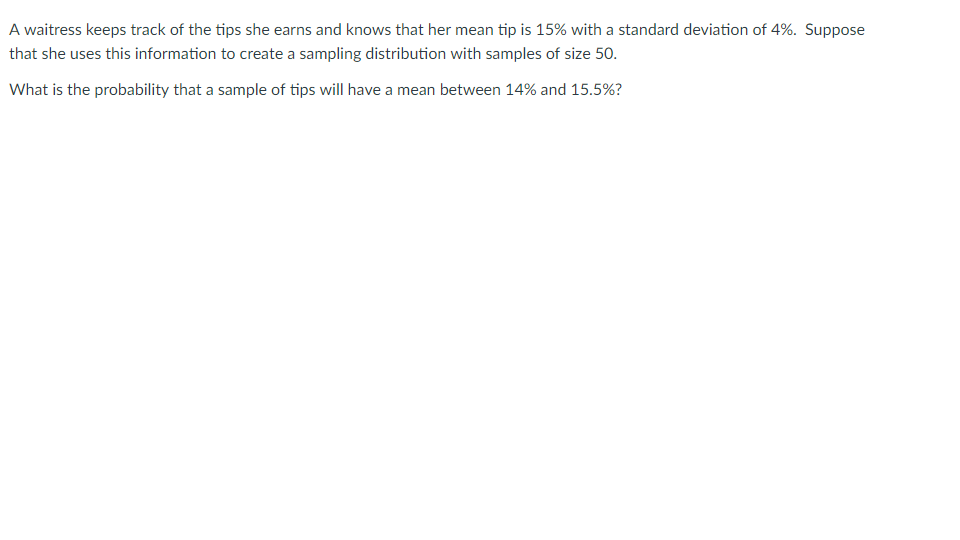# A waitress keeps track of the tips she earns and knows that her mean tip is 15% with a standard deviation of 4%. Supposethat she uses this information to create a sampling distribution with samples of size 50What is the probability that a sample of tips will have a mean between 14% and 15.5%?

Question
6 viewshelp_outlineImage TranscriptioncloseA waitress keeps track of the tips she earns and knows that her mean tip is 15% with a standard deviation of 4%. Suppose that she uses this information to create a sampling distribution with samples of size 50 What is the probability that a sample of tips will have a mean between 14% and 15.5%? fullscreen
check_circle

Step 1

We have been given that a waitress keeps a track of the tips that she earns and knows that her mean tip is 15% with a standard deviation of 4%. She uses this information to create a sampling distribution with samples of size 50. We need to determine the probability that a sample of tips will have a mean between 14% and 15.5%. We will use normal distribution to determine the required probability.

Our first step is to find the z scores corresponding to the values x=14% and x = 15.5%.

Step 2

We will use the formula for z score as shown below to find the values of z scores corresponding to x = 14% and x = 15.5%

Step 3

z score corresponding to x = 1...

### Want to see the full answer?

See Solution

#### Want to see this answer and more?

Solutions are written by subject experts who are available 24/7. Questions are typically answered within 1 hour.*

See Solution
*Response times may vary by subject and question.
Tagged in

### Statistics